﻿ Statistical Analysis on the Rate of Kidney (Renal) Failure

### Statistical Analysis on the Rate of Kidney (Renal) Failure

Vishwa Nath Maurya, Vijay Vir Singh, Madaki Umar YusufOPEN ACCESSPEER-REVIEWED

## Statistical Analysis on the Rate of Kidney (Renal) Failure

Vishwa Nath Maurya1,, Vijay Vir Singh2, Madaki Umar Yusuf2

1Professor & Head, Department of Mathematics & Statistics, the University of Fiji, Fiji

2Department of Mathematics and Statistics, Yobe State University, Damaturu, Nigeria

### Abstract

This paper is based on statistical analysis of rate of kidney renal failure taking into account that the variables of interest are sex and age group. The nature of the data used herein is secondary data, which was obtained from University of Maiduguri Teaching Hospital (UMTH) medical record for consecutive ten (10) years (1998-2007), while monthly reported cases was collected and analyzed. Our present study has been carried out in order to determine whether the effect of renal failure depends on age and sex, and to look at the prevalence of kidney (renal) failure, over the period of study. Appropriate statistical techniques have been used to test the difference of means (t-test) and contingency table (x2 -test), based on the analysis of results. The analysis has been done for significant at 5% level of significance. The empirical results are obtained from the tests of two different means which reveal that there is a significant difference in the prevalent of renal failure between male and female. Resultantly, the impact of kidney renal failure has been focused both on two parameters of age and sex. Finally, some significant suggestions based on our empirical results and observations have also been proposed for preventing kidney renal failure and future scope of present study.

• Maurya, Vishwa Nath, Vijay Vir Singh, and Madaki Umar Yusuf. "Statistical Analysis on the Rate of Kidney (Renal) Failure." American Journal of Applied Mathematics and Statistics 2.6A (2014): 6-12.
• Maurya, V. N. , Singh, V. V. , & Yusuf, M. U. (2014). Statistical Analysis on the Rate of Kidney (Renal) Failure. American Journal of Applied Mathematics and Statistics, 2(6A), 6-12.
• Maurya, Vishwa Nath, Vijay Vir Singh, and Madaki Umar Yusuf. "Statistical Analysis on the Rate of Kidney (Renal) Failure." American Journal of Applied Mathematics and Statistics 2, no. 6A (2014): 6-12.

 Import into BibTeX Import into EndNote Import into RefMan Import into RefWorks

### 1. Introduction

A kidney renal failure is a serious disease, which has major impact on life and can be accidentally fatal; several studies have demonstrated the high incidence of renal failure, which are of two types i.e. acute and chronic renal failures. Kidney disease is an important public health issue. It is common and the prevalence increases with age, which means that the disease burden will increase with our aging population. Chronic kidney disease is an independent risk factor for other diseases, particularly cardiovascular disease. It often coexists with other cardiovascular conditions meaning that it needs to be managed alongside other diseases and risk factors such as diabetes and hypertension as well as the social needs that come with frailty and multiple conditions. In a minority of cases, chronic kidney disease progresses to end stage renal disease, which may require renal replacement therapy. This progression and the risks of other vascular events, such as stroke and heart failure can be reduced if chronic kidney disease is identified and managed, early diagnosis is therefore essential. The acute renal failure (ARF) is characterized usually reversible deterioration of renal function, which develops over a period of days or week. It occurs suddenly, by causing bacterial infection, injuries, shock, congestive heart failure, drug poisoning and severed bleeding which results in uremia. A marked reduction in urine volumed is usual and the clinical features, while the rapid problems of diagnosis and management arises. Many of the disorder giving rise to acute renal failure carry high rate of mortality in human beings, but if the patients survives, then the renal function usually returns to normal or near normal. Chronic kidney disease (CKD) describes abnormal kidney function and/or structure. It is common, frequently unrecognized and often exists together with other conditions (for example, cardiovascular disease and diabetes). CKD can progress to end stage renal disease in a small but significant percentage of people . CKD is usually asymptomatic until the late stages, but it is detectable usually by measurement of serum creatinine or urine testing for protein. In the UK clinical practice has been standardized using the 4 factor Modification of Diet in Renal Disease (MDRD) equation and albumin creatinine ratio, consistent with the National Institute for Health and Clinical Excellence (NICE) guidance . Other measurement methods exist for specific indications such as the CKD-EPI equation and the Cockroft-Gault in children. The CKD-EPI equation is more accurate than the MDRD especially in categorizing CKD stages 3-5 and may be used in future CKD guidelines . There is evidence that treatment can prevent or delay the progression of CKD, reduce or prevent the development of complications and reduce the risk of cardiovascular disease. Statistical simulations techniques and sampling tests are widely used to explore significant empirical results and implications in different allied fields of biology, natural science, and life sciences. In this direction, we refer recent work of Maurya  and references therein. Literature shows that several previous researchers and authors [2, 9, 10, 11, 12] paid their attention to contribute in this connection.

### 2. Statement of the Problem

The cases of kidney renal failure over the years especially in Nigeria, has been observed to be fluctuating, despite the fact that the disease can be accidentally fatal, so therefore, renal failure may be caused by any condition, which destroys the normal structures and functions of the kidney. Obviously, for that reason it has been observed that there was a great impact in the problem due to some prevention, which arises today in the human health organization. By the aims and objectives mentioned below, one will be able to know the discrepancies arising the effects of renal failure and how it can be cured. As a result of its great economic importance, there is a need to address both educated and illiterate people so as to know the implications and protections in human societies. It is this development that prompted the desire to look at the situation morally and clearly, so as to draw a valid conclusion.

### 3. The Aim and Objectives

The aim and objectives that govern the renal failure are:

To verify whether the effect of renal failure depends on age and sex together with the objectives.

To verify whether there is any difference in the prevalence of renal failure between sexes.

To analyze, verify, recommend and conclude based on the result of the analysis made to the research on the effect of renal failure.

### 4. Research Questions

•  Does the number of renal failure increases or decreases over a period of time at different age group?

•  Does the number of renal failure depend on age and sex?

•  At what age is the renal failure more rampant and prevalent?

### 5. Research Hypothesis

1. Null hypothesis Ho: kidney (renal) failure does not depends on age and sex: Ho: u1=u2. Alternative hypothesis H1: kidney (renal) failure depends on age and sex: H1: u1#u2

2. Null hypothesis Ho: there is no significant difference in the prevalence of kidney (renal) failure between sexes: Ho: u1=u2. Alternative hypothesis: there is a significant difference in the prevalence of kidney (renal) failure between sexes: H1:u1#u2.

### 6. Significance of the Study

Study of literature shows that much work has been carried out on statistical analysis on health related issues. Apart from this major sector to the pursuance of my education it can also serve as baseline information. Researchers that may be willing to carryout relevant study in the future and also the analysis will be great significance to the University of Maiduguri Teaching Hospital and Borno State Ministry of Health in general.

### 7. Scope and Limitation of the Study

This study is limited to the number of reported cases on the rate of kidney (renal) failure at the University of Maiduguri Teaching Hospital (UMTH) for the year (1998-2007). It entails some limitation especially in the field of data collection in the study is restricted to only University of Maiduguri Teaching Hospital. Considering the wideness of this topic, the analysis is based on the ten (10) years monthly reported cases of the renal failure in Maiduguri and other towns nearby the state.

A chi-squared test also referred to as chi-square test or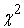test is any statistical hypothesis in which the sampling distribution of the test statistic is a chi-squared distribution when the null hypothesis is true. Also considered a chi-squared test is a test in which this is asymptotically true, meaning that the sampling distribution (if the null hypothesis is true) can be made to approximate a chi-squared distribution as closely as desired by making the sample size large enough. He computed the sampling distribution of the sample variance of a normal population. Thus in German this was traditionally known as the Helmertsche ("Helmertian") or "Helmert distribution". The name "chi-squared" ultimately derives from Pearson's shorthand for the exponent in a multivariate normal distribution with the Greek letter Chi, writing -½χ² for what would appear in modern notation as -½xTΣ-1x (Σ being the covariance matrix). The idea of a family of "chi-squared distributions" is however not due to Pearson but arose as a further development due to Fisher in the 1920s.

### 8. Calculating the Test-Statistic

The value of the test-statistic is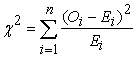Where= Pearson's cumulative test statistic, which asymptotically approaches adistribution.= an observed frequency;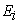= an expected (theoretical) frequency, asserted by the null hypothesis;

n= the number of cells in the table.

### 9. Assumptions for the Chi-Square Distribution

The data obtained from a random samples

The expected value in each cell must be at least 5 or more.

### 10. Contingency Table

A contingency table (also referred to as cross tabulation or cross tab) is a type of table in a matrix format that displays the (multivariate) frequency distribution of the variables. The term contingency table was first used by Karl Pearson in "On the theory of contingency and its relation to association and normal correlation", part of the Drapers' Company Research Memoirs Biometric Series I published in 1904. A crucial problem of multivariate statistics is finding (direct-) dependence structure underlying the variables contained in high dimensional contingency tables. If some of the conditional independences are revealed, then even the storage of the data can be done in a smarter way. In order to do this one can use information theory concepts, which gain the information only from the distribution of probability, which can be expressed easily from the contingency table by the relative frequencies.

The contingency table is given as follows;

### 11. Measures of Association

The degree of association between the two variables can be assessed by a number of coefficients: the simplest is the phi coefficient defined byWhere χ2 is derived from Pearson's chi-squared test, and N is the grand total of observations. φ varies from 0 (corresponding to no association between the variables) to 1 or -1 (complete association or complete inverse association). This coefficient can only be calculated for frequency data represented in 2 x 2 tables. φ can reach a minimum value -1.00 and a maximum value of 1.00 only when every marginal proportion is equal to. 50 (and two diagonal cells are empty). Otherwise, the phi coefficient cannot reach those minimal and maximal values.

Alternatives include the tetrachoric correlation coefficient (also only applicable to 2 × 2 tables), the contingency coefficient C, and Cramér's V. C suffers from the disadvantage that it does not reach a maximum of 1 or the minimum of -1; the highest it can reach in a 2 x 2 table is. 707; the maximum it can reach in a 4 × 4 table is 0.870. It can reach values closer to 1 in contingency tables with more categories. It should, therefore, not be used to compare associations among tables with different numbers of categories. Moreover, it does not apply to asymmetrical tables (those where the numbers of row and columns are not equal).

The formulae for the C and V coefficients are: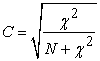and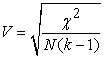k being the number of rows or the number of columns, whichever is less.

C can be adjusted so it reaches a maximum of 1 when there is complete association in a table of any number of rows and columns by dividing C by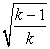(recall that C only applies to tables in which the number of rows is equal to the number of columns and therefore equal to k).

The tetrachoric correlation coefficient assumes that the variable underlying each dichotomous measure is normally distributed. The tetrachoric correlation coefficient provides "a convenient measure of [the Pearson product-moment] correlation when graduated measurements have been reduced to two categories. The tetrachoric correlation should not be confused with the Pearson product-moment correlation coefficient computed by assigning, say, values 0 and 1 to represent the two levels of each variable (which is mathematically equivalent to the phi coefficient). An extension of the tetrachoric correlation to tables involving variables with more than two levels is the polychoric correlation coefficient.

The Lambda coefficient is a measure of the strength of association of the cross tabulations when the variables are measured at the nominal level. Values range from 0 (no association) to 1 (the theoretical maximum possible association). Asymmetric lambda measures the percentage improvement in predicting the dependent variable. Symmetric lambda measures the percentage improvement when prediction is done in both directions.

The uncertainty coefficient is another measure for variables at the nominal level.

The values range from -1 (100% negative association, or perfect inversion) to +1 (100% positive association, or perfect agreement). A value of zero indicates the absence of association.

### 12. Yates's Correction for Continuity

Yates' correction for continuity (or Yates' chi-squared test) is used in certain situations when testing for independence in a contingency table. In some cases, Yates' correction may adjust too far, and so its current use is limited. Using the chi-squared distribution to interpret Pearson's chi-squared statistic requires one to assume that the discrete probability of observed binomial frequencies in the table can be approximated by the continuous chi-squared distribution. This assumption is not quite correct, and introduces some error.

To reduce the error in approximation, Frank Yates, an English statistician, suggested a correction for continuity that adjusts the formula for Pearson's chi-squared test by subtracting 0.5 from the difference between each observed value and its expected value in a 2 × 2 contingency table. This reduces the chi-squared value obtained and thus increases its p-value.

The effect of Yates' correction is to prevent overestimation of statistical significance for small data. This formula is chiefly used when at least one cell of the table has an expected count smaller than 5. Unfortunately, Yates' correction may tend to overcorrect. This can result in an overly conservative result that fails to reject the null hypothesis when it should (a type II error). So it is suggested that Yates' correction is unnecessary even with quite low sample sizes , such as: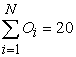The following is Yates' corrected version of Pearson's chi-squared statistic:where:

Oi = an observed frequency

Ei = an expected (theoretical) frequency, asserted by the null hypothesis

N = number of distinct events

### 13. Student's T-Test

A t-test is any statistical hypothesis test in which the test statistic follows a Student's t distribution if the null hypothesis is supported. It can be used to determine if two sets of data are significantly different from each other, and is most commonly applied when the test statistic would follow a normal distribution if the value of a scaling term in the test statistic were known. When the scaling term is unknown and is replaced by an estimate based on the data, the test statistic (under certain conditions) follows a Student's t distribution. The t-statistic was introduced in 1908 by William Sealy Gosset, a chemist working for the Guinness brewery in Dublin, Ireland ("Student" was his pen name). Gosset had been hired due to Claude Guinness's policy of recruiting the best graduates from Oxford and Cambridge to apply biochemistry and statistics to Guinness's industrial processes . Gosset devised the t-test as a cheap way to monitor the quality of stout. The t-test work was submitted to and accepted in the journal Biometrika, the journal that Karl Pearson had co-founded and was the Editor-in-Chief; the article was published in 1908. Company policy at Guinness forbade its chemists from publishing their findings, so Gosset published his mathematical work under the pseudonym "Student". Actually, Guinness had a policy of allowing technical staff leave for study (so-called study leave), which Gosset used during the first two terms of the 1906-1907 academic year in Professor Karl Pearson's Biometric Laboratory at University College London. Gosset's identity was then known to fellow statisticians and the Editor-in-Chief Karl Pearson. It is not clear how much of the work Gosset performed while he was at Guinness and how much was done when he was on study leave at University College London. Among the most frequently used t-tests are:

A one-sample location test of whether the mean of a normally distributed population has a value specified in a null hypothesis.

A two-sample location test of the null hypothesis that the means of two normally distributed populations are equal. All such tests are usually called Student's t-tests, though strictly speaking that name should only be used if the variances of the two populations are also assumed to be equal; the form of the test used when this assumption is dropped is sometimes called Welch's t-test. These tests are often referred to as "unpaired" or "independent samples" t-tests, as they are typically applied when the statistical units underlying the two samples being compared are non-overlapping. In testing the null hypothesis that the population mean is equal to a specified value μ0, one uses the statisticWhereis the sample mean, s is the sample standard deviation of the sample and n is the sample size. The degrees of freedom used in this test are n − 1.

### 14. Independent Two-Sample T-Test

14.1. Equal Sample Sizes, Equal Variance

This test is only used when both:

the two sample sizes (that is, the number, n, of participants of each group) are equal;

it can be assumed that the two distributions have the same variance.

Violations of these assumptions are discussed below.

The t statistic to test whether the means are different can be calculated as follows: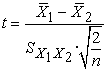whereHere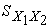is the grand standard deviation (or pooled standard deviation), 1 = group one, 2 = group two. The denominator of t is the standard error of the difference between two means.

For significance testing, the degrees of freedom for this test is 2n - 2 where n is the number of participants in each group.

14.2. Unequal Sample Sizes, Equal Variance

This test is used only when it can be assumed that the two distributions have the same variance. (When this assumption is violated, see below.) The t statistic to test whether the means are different can be calculated as follows: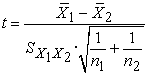Where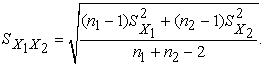Note that the formulae above are generalizations of the case where both samples have equal sizes (substitute n for n1 and n2).is an estimator of the common standard deviation of the two samples: it is defined in this way so that its square is an unbiased estimator of the common variance whether or not the population means are the same. In these formulae, n = number of participants, 1 = group one, 2 = group two. n − 1 is the number of degrees of freedom for either group, and the total sample size minus two (that is, n1 + n2 − 2) is the total number of degrees of freedom, which is used in significance testing.

14.3. Unequal (or Equal) Sample Sizes, Unequal Variances

This test, also known as Welch's t-test, is used only when the two population variances are not assumed to be equal (the two sample sizes may or may not be equal) and hence must be estimated separately. The t statistic to test whether the population means are different is calculated as: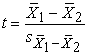Where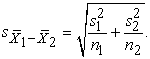Here s2 is the unbiased estimator of the variance of the two samples, ni = number of participants in group i, i=1 or 2. Note that in this case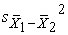is not a pooled variance. For use in significance testing, the distribution of the test statistic is approximated as an ordinary Student's t distribution with the degrees of freedom calculated using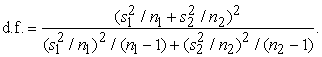This is known as the Welch–Satterthwaite equation. The true distribution of the test statistic actually depends (slightly) on the two unknown population variances (see Behrens–Fisher problem).

### 15. Dependent T-Test for Paired Samples

This test is used when the samples are dependent; that is, when there is only one sample that has been tested twice (repeated measures) or when there are two samples that have been matched or "paired". This is an example of a paired difference test.For this equation, the differences between all pairs must be calculated. The pairs are either one person's pre-test and post-test scores or between pairs of persons matched into meaningful groups (for instance drawn from the same family or age group: see table). The average (XD) and standard deviation (sD) of those differences are used in the equation. The constant μ0 is non-zero if you want to test whether the average of the difference is significantly different from μ0. The degree of freedom used is n − 1. We remark here that the statistical tests and techniques applied here are analyzed and used by Maurya et al. [5, 7] in their recent research work.

### 16. Assumptions for The Student's T-Test Distribution

Most t-test statistics have the form t = Z/s, where Z and s are functions of the data. Typically, Z is designed to be sensitive to the alternative hypothesis (i.e., its magnitude tends to be larger when the alternative hypothesis is true), whereas s is a scaling parameter that allows the distribution of t to be determined.

As an example, in the one-sample t-test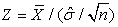, where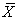is the sample mean of the data, n is the sample size, and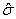is the population standard deviation of the data; s in the one-sample t-test is, whereis the sample standard deviation.

### 17. The Assumptions Underlying A T-Test Are That

Z follows a standard normal distribution under the null hypothesis

s2 follows a χ2 distribution with p degrees of freedom under the null hypothesis, where p is a positive constant

Z and s are independent.

In a specific type of t-test, these conditions are consequences of the population being studied, and of the way in which the data are sampled. For example, in the t-test comparing the means of two independent samples, the following assumptions should be met:

Each of the two populations being compared should follow a normal distribution. This can be tested using a normality test, such as the Shapiro-Wilk or Kolmogorov–Smirnov test, or it can be assessed graphically using a normal quantile plot.

If using Student's original definition of the t-test, the two populations being compared should have the same variance (testable using F test, Levene's test, Bartlett's test, or the Brown–Forsythe test; or assessable graphically using a Q-Q plot). If the sample sizes in the two groups being compared are equal, Student's original t-test is highly robust to the presence of unequal variances. Welch's t-test is insensitive to equality of the variances regardless of whether the sample sizes are similar.

The data used to carry out the test should be sampled independently from the two populations being compared. This is in general not testable from the data, but if the data are known to be dependently sampled (i.e. if they were sampled in clusters), then the classical t-tests discussed here may give misleading results.

### 18. Results and Discussions

In this part, we shall employ the use of statistical tools that we discussed earlier so as to present out data and analyzed it. The statistical tools to be applied to verify our research hypothesis, contingency table (x2 test) and test of two different means (t-test) are the tests used in the analysis.

18.1. Chi – Square Test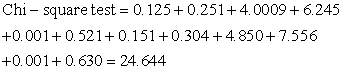DF = 5, P-value = 0.000.

From the table of chi-square test analysis, stating the hypothesis:

Null hypothesis Ho: kidney (renal) failure does not depends on age and sex: Ho: u1=u2. Alternative hypothesis H1: kidney (renal) failure depends on age and sex: H1:u1#u2

With test statistic:With (r-1)(c-1)

Where Oij = observed number of sex counts in the ith row of the jth column

Where Eij = expected number of sex counts in the ith row of the jth column

Decision criterion: reject Ho if probability < significant level otherwise reject.

From the table 1, it shows that the chi-square calculated value is 24.644 and the chi-square tabulated value at 5% level of significance, with (6-1)(2-1) = 5 is 11.070. Therefore since X2

Calculated >= X2 tabulated, we reject the null hypothesis and conclude that kidney (renal) failure depends on age and sex and therefore there is a significant differences, that is 24.644>11.070 and p-value given as 0.000 and the significant level at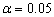. In conclusion, since p-value < significant level i.e, 0.000 < 0.05, we reject the hypothesis.

18.2. T-TEST

#### Table

From the table 2, it shows that the test of two different means, stating the hypothesis;

Null hypothesis Ho: there is no significant difference in the prevalence of kidney (renal) failure between sexes: Ho: u1=u2. Alternative hypothesis: there is a significant difference in the prevalence of kidney (renal) failure between sexes: H1:u1#u2.

18.3. Test Statistic

calculated as:WhereHere s2 is the unbiased estimator of the variance of the two samples, ni = number of participants in group i, i=1 or 2. Note that in this caseis not a pooled variance. For use in significance testing, the distribution of the test statistic is approximated as an ordinary Student's t distribution with the degrees of freedom calculated usingThe significance level at.

### 19. Decision Criterion

We reject the hypothesis if the p-value < significant level otherwise do not reject the hypothesis if t calculated >= t, n1+n2-2, atlevel of significance.

### 20. Conclusions

From the table 2, this shows that the test of two different means, that is the t-calculated value which is 3.36 and the t-tabulated value at 5% level of significance, with (10+10-2) = 18 degree of freedom is 2.01. Therefore since t-calculated > t-tabulated, we reject the null hypothesis and conclude that there is a significant differences between the male and female sexes which shows that there is a highly positive correlation between male and female sexes and therefore, that is 0.008 < 0.05, we reject the hypothesis and conclude that there is a significant difference

### 21. Coefficient of Contingency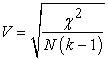Where, N = total number of observation.

The larger the value of the coefficient, the greater the degree of association. The greater the degree of association. The coefficient can take on values between zero and one.

21.1. DataThis shows that there is a weak relationship between age and sex.

### Conclusion and Recommendations

The data analyzed on monthly and yearly basis of reported cases on the rate of kidney renal failure, at the university of Maiduguri teaching hospital (UMTH) reveal that there is a little reduction in the number of patients. From the result of chi-square test (contingency table) it was observed that the average rate at which real failure affect people, the result shows that it is significant and indicates that the age of person depends on sex, at the same time the coefficient of contingency shows a weak relationship between the age and sex. Despite the fact that, the rate at which kidney (renal) failure is failed, it is observed and believed that it will have influence on its great economic importance and this shows from the test that there are a little bit decreases as a result of the improvement in treatments. Also from the test of difference of two means it was observed that the renal failure is significant difference between the male and female sexes.

From the analysis, we observed an increase and decrease at both time. So there is need for improvement especially in the area of supply of genuine drugs to the hospital and the affected patients. Since one of the analysis shows that there is a difference among the sexes at both time, then the inspectorate division of national agencies for health, drugs administrative and control should continue inspecting, advising the illiterate and educated people so as to to know the implication of the kidney diseases. Generally, government should provide more equipments and provision for more health workers and better coordination of services to professional doctors and nurses so as to take care of the affected persons in the society.

### References

  Cochran W.G.(1954), Some methods of strengthening the common x2 tests. Biometrics, 10, 417-51.In article CrossRef  Dibal, N.P (2006), Elementary Statistics, Loud Books Publishers, Konji-Bodija, Ibadan, 2nd Edition.In article  Dibal, N.P. (2006), Research Methods, books publishers, Konji-Bodija, Ibadan, 1st Edition.In article  Frank H. and Althoen, S.C. (1995), Statistics Concepts and Applications.In article  Maurya V.N., Maurya A.K. and Kaur D. (2013), A survey report on nonparametric hypothesis testing including Kruskal-Wallis ANOVA and Kolmogorov–Smirnov goodness-fit-test, International Journal of Information Technology & Operations Management, Academic and Scientific Publishing, New York, USA, Vol. 1, No. 2, pp. 29-40, ISSN: 2328-8531In article  Maurya V.N. (2013), Numerical simulation for nutrients propagation and microbial growth using finite difference approximation technique, International Journal of Mathematical Modeling and Applied Computing, Academic & Scientific Publishing, New York, USA, Vol. 1, No. 7, pp. 64-76, November, ISSN: 2332-3744In article  Maurya V.N., Maurya A.K. & Arora D.K. (2014), Elements of Advanced Probability Theory and Statistical Techniques, Scholar’s Press Publishing Co., Saarbrucken, Germany, ISBN 978-3-639-51849-8In article  Maxwell E. (1971), Analysing Qualitative Data. 4th Edition. Chapman and Hall Ltd. Library of Congress Catalog Card Number 75-10907.In article  Murray R.S, John S.R and Srinivasan, R.A (2004), Probability and Statistics, 2nd Edition.In article  Rayner J.C.W. and Best D.J. (1989), Smooth Tests of Goodness of Fit. Oxford University Press, Inc., ISBN 0-19-505610-8.In article  Urdan Timothy C. (2005), Statistics in Plain English. 2nd Edition. Lawrence Erlbaum Associates, Inc., London, UKIn article  William Mendenhall, Beaver Robert J., and Beaver Barbara M. (2003), Introduction to Probability and Statistics. Brooks/Cole, Division of Thomson Learning, Inc., 2003. ISBN 0-534-39519-8.In article